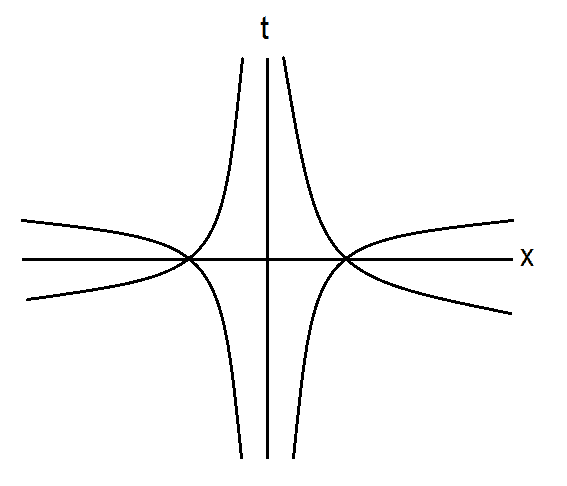# Spacetime diagram for black hole

## Homework Statement

Consider a 2D spacetime with:
## ds^2 = g_{tt}dt^2 + g_{tx}(dtdx + dxdt)##
where: ##g_{tt} = -x## and ##g_{tx}=3##

Draw a spacetime diagram showing the null geodesics, including one which passes through the origin. Then show that for a massive object, when ##x## is positive, ##x## can increase or decrease as ##v## increases, but if ##x## is negative, it may only decrease.

## Homework Equations

Null geodesic: ##ds^2 = 0##

## The Attempt at a Solution

From the above equation, we get for a null geodesic:
## 0 = g_{tt}dt^2 + g_{tx}(dtdx + dxdt)##
## x dt^2 = 3(dtdx + dxdt)##
## x dt^2 = 6dtdx## (since dt can be positive or negative)
## x dt = \pm 6dx##
## dt/dx = \pm 6/x##
## t = \pm 6 ln(x)##

and for x=0, we have an infinite slope, i.e. a straight vertical line through the origin. I'm not sure if this is right but it would give a spacetime diagram like this:

http://imgur.com/EgjKZSM
http://imgur.com/EgjKZSM

Where the null geodesics represent the path light will take. But even if this diagram is right, I'm not sure how to show the next part of the question regarding the massive object.